# Classify each of the following as a path function or a state function

Classify each of the following as a path function or a state function.

• work
• energy
• distance traveled
• enthalpy
• heat

Concepts and reason
The concept used to solve this problem is based on the thermodynamic functions.
There are two types of thermodynamic functions. First is state function and second is path function.

Fundamentals
The state functions is a function that defines a particular state. Its value does not depend on the path taken to reach a specific value.
The path function is a function whose value depends on the path taken to reach a specific value.

Part 1
The enthalpy and energy are the type of functions whose values depend on the initial and final state of the system but not on the path followed. For two states change in energy can be written as follow.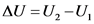Similarly, change in enthalpy can be written as follow.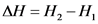Thus, their values do not depend on the path taken to reach the state.Explanation:
The state functions depends only on the initial and final states of the system but not depend upon the path it followed.

Part 2
The functions work, heat and distance travelled do not depend on initial and final states of the system but they depend on path followed between initial and final states.
If there are two paths to reach a specific value then work functions is written as follow.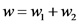Similarly, heat function is written as follow.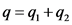And, distance travelled is written as follow.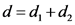So, these functions depend on the path taken to reach a specific value.Explanation:
The path functions depend on the path taken to reach a particular state of the system.